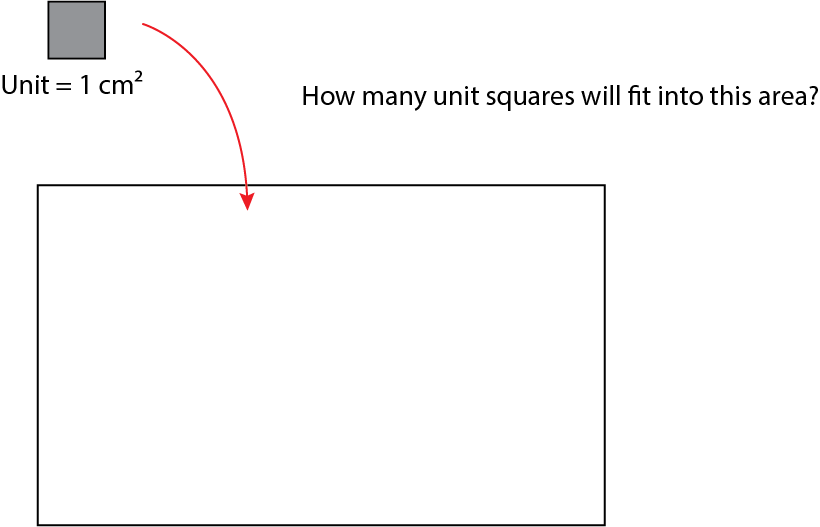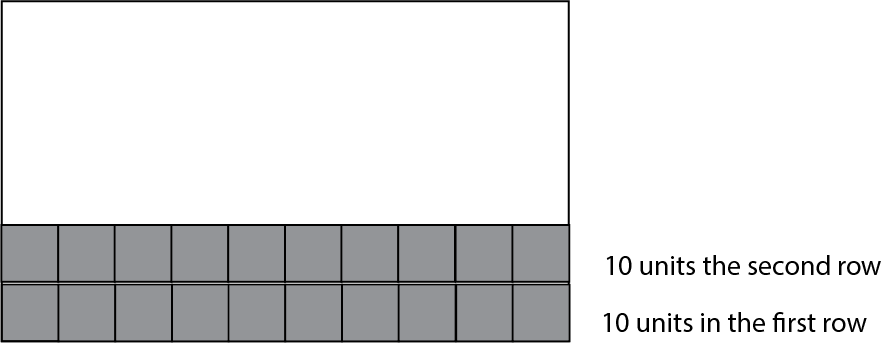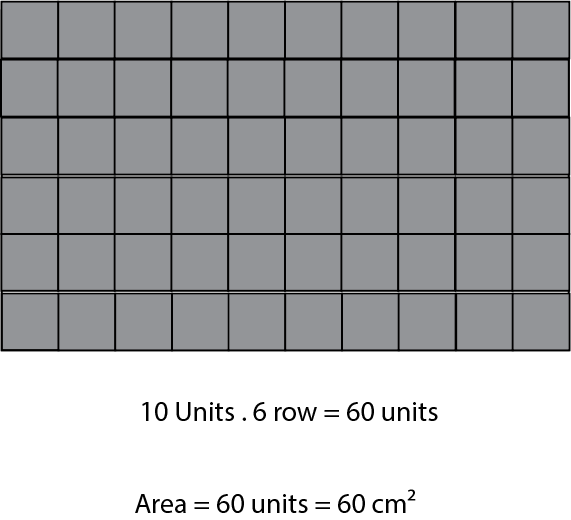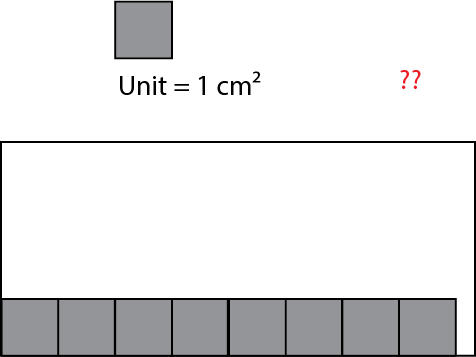### Area

Area : The space bounded by any two-dimensional shape in a plane.

Let's paint the potatoes in different shapes, then press them onto paper. The area is wherever there's paint on the paper; that's the area of your shape.

Handprint Painting

How much imprint you leave is the area of your hand.

The more you paint, the greater your area is. Area is the imprint on the plane. Area is two-dimensional and is a surface.

Painted Surface = Painted Area

# Examples

The figure shows a complete circle. If I cut it right through the middle and reposition the halves so they are back-to-back and tangent to each other, how does the area change? Does it increase, decrease, or remain the same?

It remains the same. I didn't add or remove any painted area, nor did I overlap any. The visible and tangible surface of the shape stayed the same.

# Example

Which one has a larger area ?

Answer : All of them have the same area; each consists of 4 units.

# Which one has a larger area ?

If you look closely at the surfaces, you can see that the area of the first shape, which is the circle, is larger.

I hope you have understood the concept of area.

# Measuring the area, How large is our area?

We use squares to determine how much the area is. Why do we measure area with squares ?

Area measurement units can vary based on geographical location, purpose of use, and system of measurement preference. Here are some common units for measuring area:

International System (SI) Units:

Square millimeter (mm²)
Square centimeter (cm²)
Square decimeter  (dm²)
Square meter (m²)
Square decameter (dam²)
Square hectometer (hm²)
Square kilometer (km²)

The units like square decameter and square hectometer are less commonly used in practice, but they are part of the metric system's logical progression.

Imperial/US Customary System:

Square inch (in² or square inches)
Square foot (ft² or square feet)
Square yard (yd² or square yards)
Square rod (or square pole or square perch)
Acre
Square mile (mi² or square miles)

While square rod, pole, or perch are traditional units, they are less commonly used in modern contexts compared to square inch, foot, yard, acre, and mile.

In addition to these, there are many different area units historically or regionally used. It's essential to pay attention to the units used when measuring or evaluating the size of an area.

#CalculateIn this example, our units fit perfectly into the shape. What if they don't? What do we need to do?For instance, if you measured a length and it's slightly more than 5 cm but not quite 6 cm, what do we do? We continue measuring with smaller units, like millimeters. Similarly, here we can use smaller unit squares.

You have to cover the entire area with squares; that's what measuring area means

# What happens if our area cannot be completely filled with squares?

Sometimes, due to the structure of the shape, filling it with squares can be really challenging. For instance, shapes with slanted edges or forms like circles. In such cases, what should we do? We can resort to what we call indirect measurement, where we deduce the area through calculations.

When we divide a square from corner to corner, we obtain two equal parts, and we frequently use this property. This isn't limited only to squares; it's also valid for rectangular areas (both squares and rectangles).

For example, to calculate the area of a triangle, we can calculate the area of a rectangle and then divide it by 2.
We will learn more logical operations in calculating the areas of shapes.

# Why do we use squares to measure area?

The calculation would really be challenging.

What happens if we use a circle?

• When you use squares to measure an area, you can easily count them or use mathematical formulas to determine the total area
• Additionally, squares can be closely packed without any gaps, unlike other shapes such as circles, which would leave spaces in between when used to measure area. Using squares ensures a more accurate representation of the space being measured